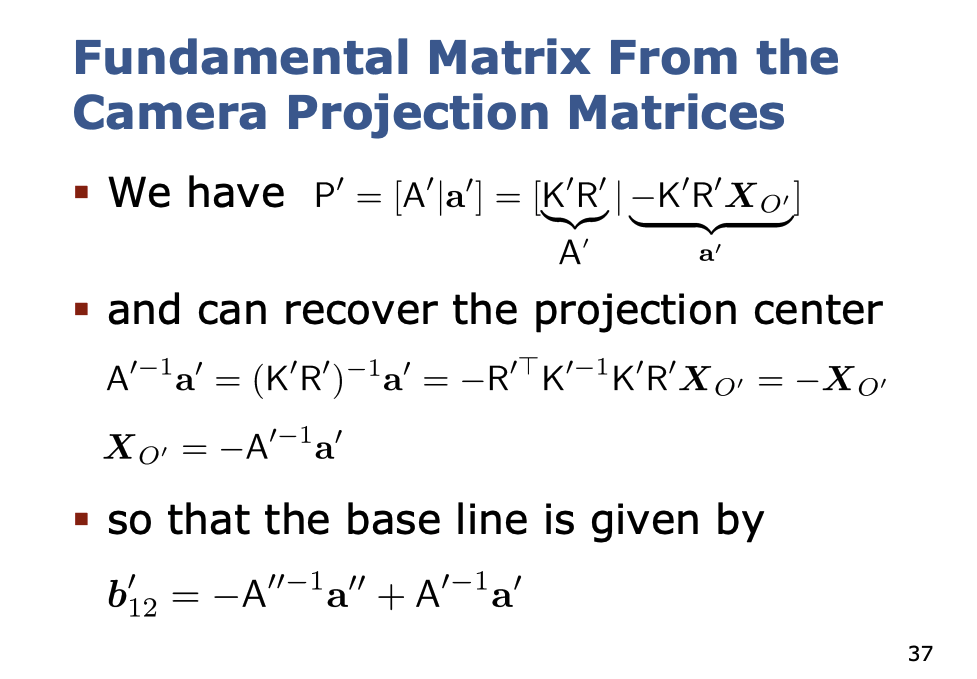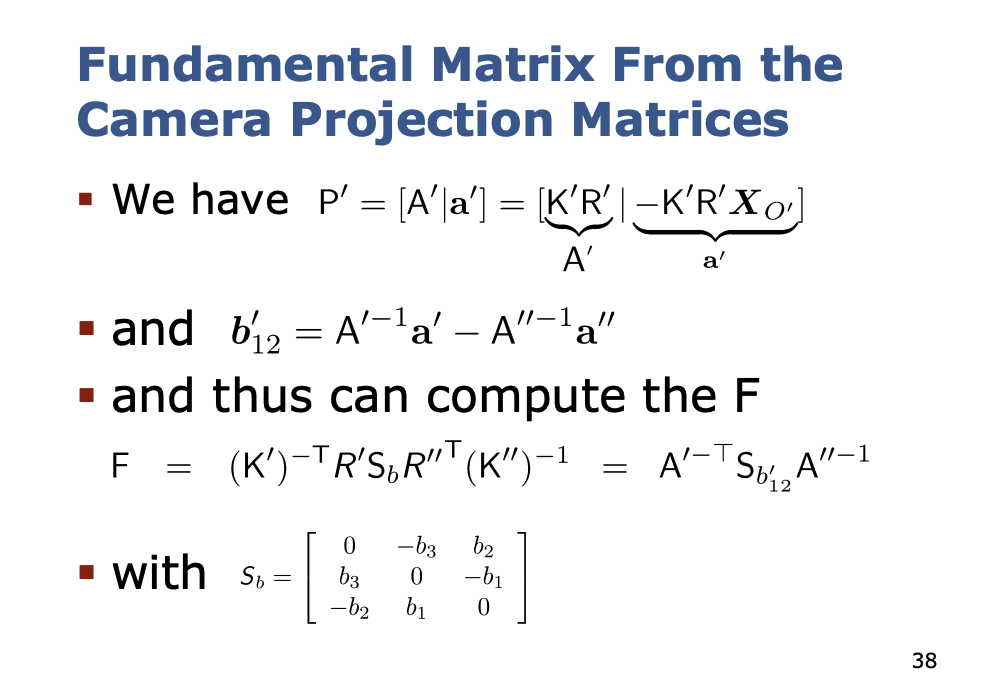# Relative Orientation §

Learned through Cyrill Stachniss.

Solved through the 5 point algorithm.

Outlier eliminated through RANSAC.

Just knowing the point correspondence, we can estimate where the camera is in the world.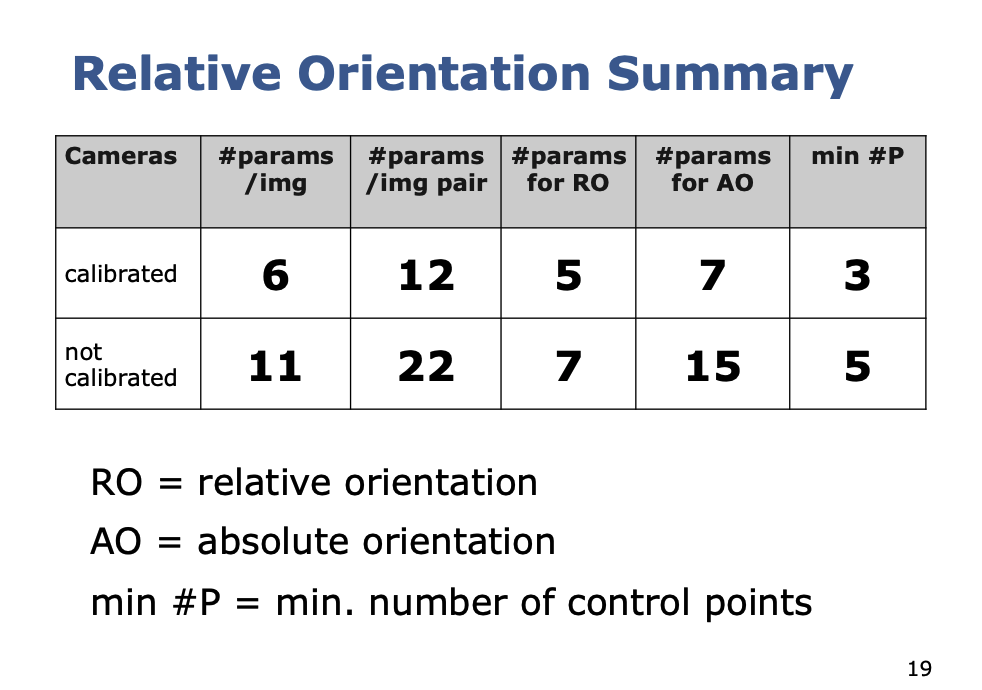Coplanarity Constraint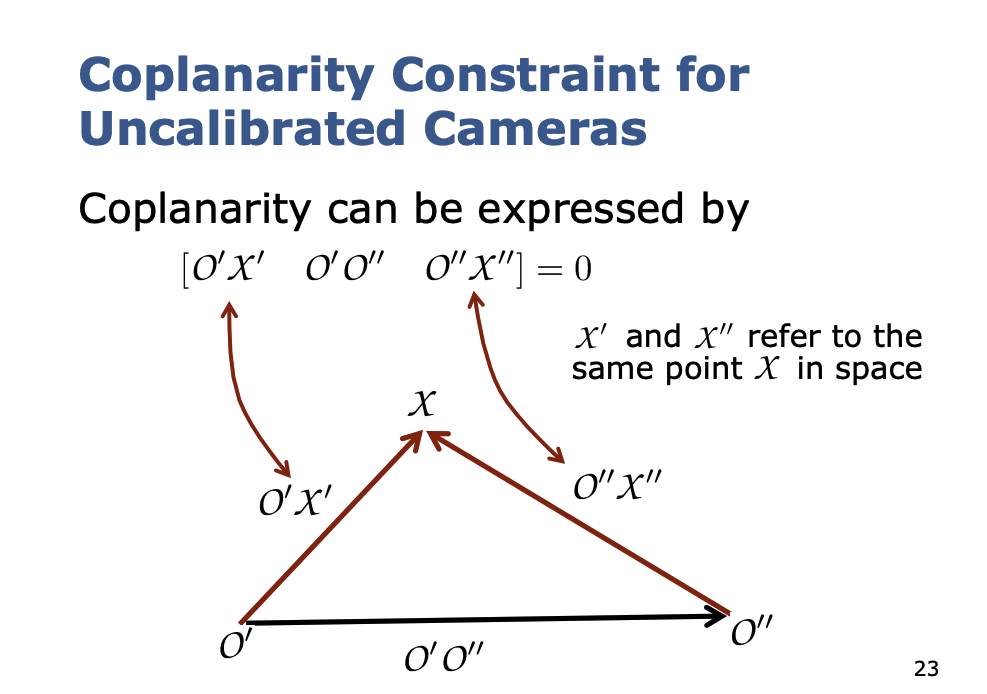We can see that all three vectors are coplanar

So that is the coplanarity constraint. We can rewrite this coplanarity constraint.

What is the point of the coplanarity constraint?

We can exploit this constraint to solve later. Because we know that the coplanarity constraint holds, all three vectors are on the same plane.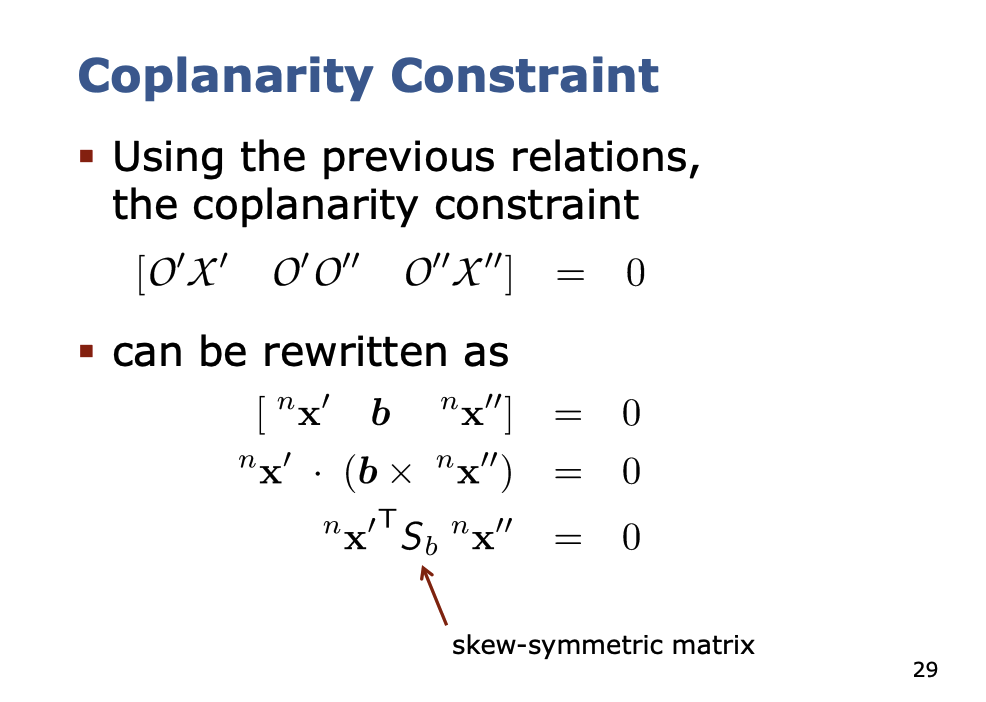Where the base vector directly results from the coordinates of the projection centers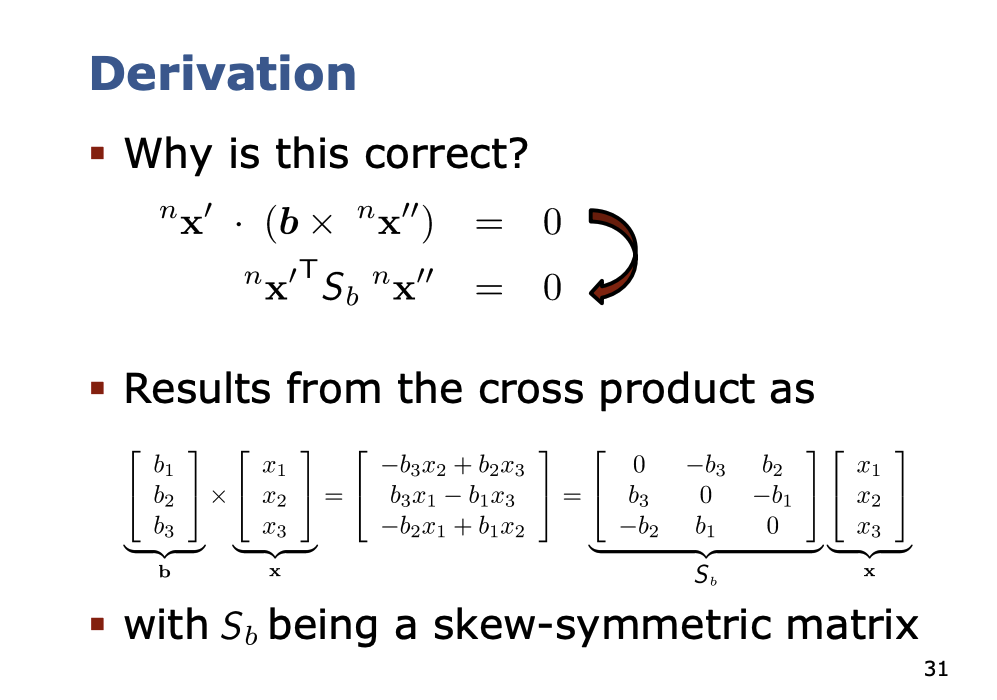Rewriting the coplanarity constraint:

The matrix is the Fundamental Matrix (for uncalibrated cameras):

It allow for expressing the coplanarity constraint by

The fundamental matrix is the matrix that fulfills the equation for corresponding points

The fundamental matrix contains the all the available information about the relative orientation of two images from uncalibrated camera.